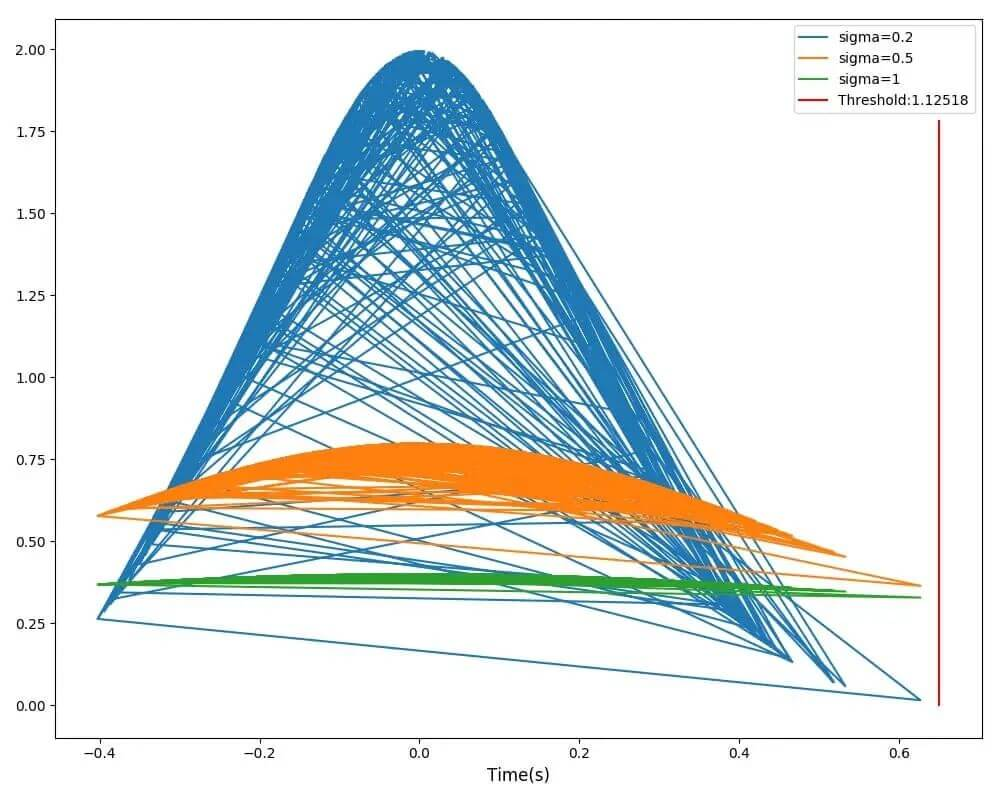# Python 函数耗时异常自动化监控实战教程

### 一、性能数据函数耗时采集及可视化报表生成

#### 1. 性能数据文件保存（cProfile）

```import cProfile
# 程序开始的时候打开数据采集开关
pr = cProfile.Profile()
pr.enable()

# 在程序运行结束的时候dump性能数据到指定路径文件中，profliePath为保存文件的绝对路径参数
pr.dump_stats(profliePath)```

#### 2. 详细性能数据读取查看

```import pstats
# 读取性能数据
pS = pstats.Stats(profliePath)
# 根据函数自身累计耗时做排序
pS.sort_stats('tottime')
# 打印所有耗时函数信息
pS.print_stats()
print_stats()输出示例：
79837 function calls (75565 primitive calls) in 37.311 seconds
Ordered by: internal time
ncalls  tottime  percall  cumtime  percall  filename:lineno(function)
2050    30.167    0.015   30.167    0.015  {time.sleep}
16     6.579    0.411    6.579    0.411  {select.select}
1     0.142    0.142    0.142    0.142  {method 'do_handshake' of '_ssl._SSLSocket' objects}
434     0.074    0.000    0.074    0.000  {method 'read' of '_ssl._SSLSocket' objects}
1     0.062    0.062    0.062    0.062  {method 'connect' of '_socket.socket' objects}
37     0.046    0.001    0.046    0.001  {posix.read}
14     0.024    0.002    0.024    0.002  {posix.fork}```

• ncalls  函数被调用次数（只有一个数字时表示不存在递归，有斜杠分割数字时，后面的数字表示非递归调用的次数）
• tottime  函数总计运行时间，不包括子函数调用时间
• percall  函数运行一次的平均时间，等于tottime/ncalls
• cumtime 函数总计运行时间，包括子函数调用时间
• percall  函数运行一次的平均时间，等于cumtime/ncalls
• filename:lineno(function) 函数所在的文件名，函数的行号，函数名或基础框架函数类

```# func————filename:lineno(function)
# cc ———— call count，调用次数
# nc ———— ncalls
# tt ———— tottime
# ct ———— cumtime
# callers ———— 调用堆栈数组，每项数据包括了func, (cc, nc, tt, ct) 字段
for index in range(len(pS.fcn_list)):
func = pS.fcn_list[index]
cc, nc, tt, ct, callers = pS.stats[func]
print cc, nc, tt, ct, func, callers
for func, (cc, nc, tt, ct) in callers.iteritems():
print func，cc, nc, tt, ct```

#### 二、生成函数调用栈结构图（gprof2dot）教程

gprof2dot脚本把gprof或callgrind分析获得的信息，转化成一个以DOT语言描述的程序调用有向图对象，再通过Graphviz将DOT有向图对象渲染成图片，这样就可以很直观地看出整个程序的调用栈，包括函数所在的类和行数、耗时占比、函数递归次数、以及被调用的次数。

`brew install graphviz`

```import os
# 获取当前gprof2dot.py脚本路径
gprof2dotPath = os.path.join(os.path.dirname(os.path.abspath(__file__)), 'gprof2dot.py')
# 函数调用栈结构图保存文件名路径，这边使用生成PNG图片结果
dumpProfPath = profliePath.replace("stats", "png")
dumpCmd = "python %s -f pstats %s | dot -Tpng -o %s" % (gprof2dotPath, profliePath, dumpProfPath)
os.popen(dumpCmd)```

### 三、性能分析及优化实战

• 去除多余的逻辑：去除冗余代码
• 优化递归函数：加日志打印递归时候的各个参数，如果发现很多参数都是重复的，可以加缓存，避免多余的递归消耗。
• 归并通用逻辑调用：一个函数多次调用同一个子函数获取参数，查看这个子函数的调用是否可以进行整合归并，避免多余的函数调用。
• 通过上下文环境判断测试程序的初始化是否必要，非必要情况下不进行测试环境的重置操作。

### 四、耗时异常自动化监控

• 基于统计和概率模型：主要是对数据的分布做出假设，并找出假设下所定义的“异常”；
• 线性模型：主要思想是通过线性方法找到合适的低维子空间使得异常点在其中区别于正常点；
• 基于距离：这种方法认为异常点距离正常点比较远，通过比较数据点之间的距离区分异常点；
• 基于密度：由于数据分布不均匀，绝对距离无法衡量数据点之间相对远近时，用局部密度表示数据点的异常情况；
• 基于聚类：将数据点聚类，不属于任何簇、距离最近的簇较远、稀疏聚类里的点认为是异常点；
• 基于树：通过划分子空间构建树模型寻找异常点。

Python中用于判断数据是否符合正态分布的代码如下，当pvalue值大于0.05时为正态分布，dataList是耗时数组数据：

```from scipy import stats
import numpy
percallMean = numpy.mean(dataList) # 计算均值
# percallVar = numpy.var(dataList) # 求方差
percallStd = numpy.std(dataList) # 计算标准差
kstestResult = stats.kstest(dataList, 'norm', (percallMean, percallStd))
# 当pvalue值大于0.05为正态分布
if kstestResult > 0.05:
pass```

#### 2. Turkey 箱型图方案

```import numpy
percallMean = numpy.mean(dataList)  # 计算均值
boxplotQ1 = numpy.percentile(dataList, 25)
boxplotQ2 = numpy.percentile(dataList, 75)
boxplotIQR = boxplotQ2 - boxplotQ1
upperLimit =  boxplotQ2 + 1.5 * boxplotIQR```

```# coding=utf-8
import os
import pstats
import matplotlib
matplotlib.use('Agg')
import matplotlib.pyplot as plt
import traceback
from scipy import stats
import numpy

"""

"""
def dataSummary(array, fileName, fcn, percall):
(funcPath, line, func) = fcn
exists = False
for item in array:
if item["func"] == func and item["funcPath"] == funcPath and item["line"] == line:
exists = True
item["cost"].append({
"percall": percall,
"fileName": fileName
})
if not exists:
array.append({
"func": func,
"funcPath": funcPath,
"line": line,
"cost": [{
"percall": percall,
"fileName": fileName
}]
})

"""

"""
def gaussian(x, mu, delta):
exp = numpy.exp(- numpy.power(x - mu, 2) / (2 * numpy.power(delta, 2)))
c = 1 / (delta * numpy.sqrt(2 * numpy.pi))
return c * exp

"""

"""
for path, dir_list, file_list in os.walk(statFolder, "r"):
for fileName in file_list:
if fileName.find(".stats") > 0:
fileAbsolutePath = os.path.join(path, fileName)
pS = pstats.Stats(fileAbsolutePath)
# 先对耗时数据从大到小进行排序
pS.sort_stats('cumtime')
# pS.print_stats()
# 统计前100条耗时数据
for index in range(100):
fcn = pS.fcn_list[index]
(funcPath, line, func) = fcn
# cc ———— call count，调用次数
# nc ———— ncalls，调用次数（只有一个数字时表示不存在递归；有斜杠分割数字时，后面的数字表示非递归调用的次数）
# tt ———— tottime，函数总计运行时间，除去函数中调用的子函数运行时间
# ct ———— cumtime，函数总计运行时间，含调用的子函数运行时间
cc, nc, tt, ct, callers = pS.stats[fcn]
# print fileName, func, cc, nc, tt, ct, callers
percall = ct / nc
# 只统计单次函数调用大于1毫秒的数据
if percall >= 0.001:
dataSummary(filterData, fileName, fcn, percall)

"""

"""
def drawGaussian(func, line, percallMean, threshold, percallList, dumpFolder):
plt.title(func)
plt.figure(figsize=(10, 8))
for delta in [0.2, 0.5, 1]:
gaussY = []
gaussX = []
for item in percallList:
# 这边为了呈现正态曲线效果，减去平均值
gaussX.append(item - percallMean)
y = gaussian(item - percallMean, 0, delta)
gaussY.append(y)
plt.plot(gaussX, gaussY, label='sigma={}'.format(delta))
# 绘制水位线
plt.plot([threshold - percallMean, threshold - percallMean], [0, 5 * gaussian(percallMean, 0, 1)], color='red',
linestyle="-", label="Threshold:" + str("%.5f" % threshold))
plt.xlabel("Time(s)", fontsize=12)
plt.legend()
plt.tight_layout()
# 可能不同类中包含相同的函数名，加上行数参数避免覆盖
imagePath = dumpFolder + "cost_%s_%s.png" % (func, str(line))
plt.savefig(imagePath)

"""

"""
def drawCurve(func, line, percallList, dumpFolder):
boxplotQ1 = numpy.percentile(percallList, 25)
boxplotQ2 = numpy.percentile(percallList, 75)
boxplotIQR = boxplotQ2 - boxplotQ1
upperLimit = boxplotQ2 + 1.5 * boxplotIQR
# 不符合正态分布，绘制波动曲线
timeArray = [i for i in range(len(percallList))]
plt.title(dataItem["func"])
plt.figure(figsize=(10, 8))
# 绘制水位线
plt.plot([0, len(percallList)], [upperLimit, upperLimit], color='red', linestyle="-",
label="Threshold:" + str("%.5f" % upperLimit))
plt.plot(timeArray, percallList, label=dataItem["func"] + "_" + str(dataItem["line"]))
plt.ylabel("Time(s)", fontsize=12)
plt.legend()
plt.tight_layout()
imagePath = dumpFolder + "cost_%s_%s.png" % (func, str(line))
plt.savefig(imagePath)

if __name__ == "__main__":
try:
chartFolder = statFolder + "chart/"
if not os.path.exists(chartFolder):
os.mkdir(chartFolder)
filterData = []
for dataItem in filterData:
percallList = map(lambda x: x["percall"], dataItem["cost"])
func = dataItem["func"]
line = dataItem["line"]
# 样本个数大于20才进行绘制
if len(percallList) > 20:
percallMean = numpy.mean(percallList) # 计算均值
# percallVar = numpy.var(percallMap) # 求方差
percallStd = numpy.std(percallList)  # 计算标准差
# pvalue值大于0.05为正太分布
kstestResult = stats.kstest(percallList, 'norm', (percallMean, percallStd))
print "percallStd:%s, pvalue:%s" % (percallStd, kstestResult)
# 符合正态分布绘制分布曲线
if kstestResult > 0.05:
threshold = percallMean + 3 * percallStd
drawGaussian(func, line, percallMean, threshold, percallList, chartFolder)
else:
drawCurve(func, line, percallList, chartFolder)
else:
pass
except Exception:
print 'exeption:' + traceback.format_exc()```函数耗时曲线及Turkey箱型图阈值示例

​Python实用宝典 ( pythondict.com )